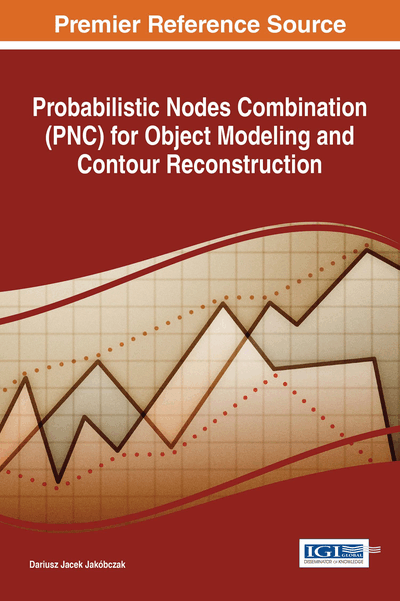# Introduction: MHR Method

DOI: 10.4018/978-1-5225-2531-8.ch001
Available
\$37.50
No Current Special Offers

## Abstract

Computer vision needs suitable methods of shape representation and contour reconstruction. One of them, invented by the author and called method of Hurwitz-Radon Matrices (MHR), can be used in representation and reconstruction of shapes of the objects in the plane. Proposed method is based on a family of Hurwitz-Radon (HR) matrices. The matrices are skew-symmetric and possess columns composed of orthogonal vectors. 2D shape is represented by the set of successive nodes. It is shown how to create the orthogonal and discrete OHR operator and how to use it in a process of shape representation and reconstruction. Contour of the object, represented by successive contour points, consists of information which allows us to describe many important features of the object as shape coefficients. 2D curve modeling is a basic subject in many branches of industry and computer science.
Chapter Preview
Top

## Introduction

Method of Hurwitz-Radon Matrices (MHR), invented by the author, can be applied in reconstruction and interpolation of curves in the plane. The method is based on a family of Hurwitz-Radon (HR) matrices. The matrices are skew-symmetric and possess columns composed of orthogonal vectors. The operator of Hurwitz-Radon (OHR), built from these matrices, is described. Author explains how to create the orthogonal and discrete OHR and how to use it in a process of curve interpolation and two-dimensional data modeling. Proposed method needs suitable choice of nodes, i.e. points of the 2D curve to be interpolated or extrapolated: nodes should be settled at each extremum (minimum or maximum) of one coordinate and at least one point between two successive local extrema, and nodes should be monotonic in one of coordinates (for example equidistance). Created from the family of N-1 HR matrices and completed with the identical matrix, system of matrices is orthogonal only for vector spaces of dimensions N = 1, 2, 4 or 8. Orthogonality of columns and rows is very important and significant for stability and high precision of calculations. MHR method is modeling the curve point by point without using any formula of function. Main features of MHR method are: accuracy of curve reconstruction depending on number of nodes and method of choosing nodes, interpolation of L points of the curve is connected with the computational cost of rank O(L), MHR interpolation is not a linear interpolation (Ullman & Basri, 1991). The problem of curve length estimation is also considered. Algorithm of MHR method and the examples of data extrapolation are described. Value anticipation is the crucial feature in risk analyzing and decision making. Risk analysis needs suitable methods of data extrapolation and decision making. Proposed method of Hurwitz-Radon Matrices (MHR) can be used in extrapolation and interpolation of curves in the plane. For example quotations from the Stock Exchange, the market prices or rate of a currency form a curve. This chapter contains the way of data anticipation and extrapolation via MHR method and decision making: to buy or not, to sell or not. Proposed method is based on a family of Hurwitz-Radon (HR) matrices. The matrices are skew-symmetric and possess columns composed of orthogonal vectors. The operator of Hurwitz-Radon (OHR), built from these matrices, is described. Two-dimensional data are represented by the set of curve points. It is shown how to create the orthogonal and discrete OHR and how to use it in a process of data foreseeing and extrapolation. MHR method is interpolating and extrapolating the curve point by point without using any formula or function.

Computer vision needs suitable methods of shape representation and contour reconstruction. One of them, invented by the author and called method of Hurwitz-Radon Matrices (MHR), can be used in representation and reconstruction of shapes of the objects in the plane. Proposed method is based on a family of Hurwitz-Radon (HR) matrices. The matrices are skew-symmetric and possess columns composed of orthogonal vectors. 2D shape is represented by the set of successive nodes. It is shown how to create the orthogonal and discrete OHR operator and how to use it in a process of shape representation and reconstruction. Then MHR method is generalized to Probabilistic Nodes Combination (PNC) method.

## Complete Chapter List

Search this Book:
Reset